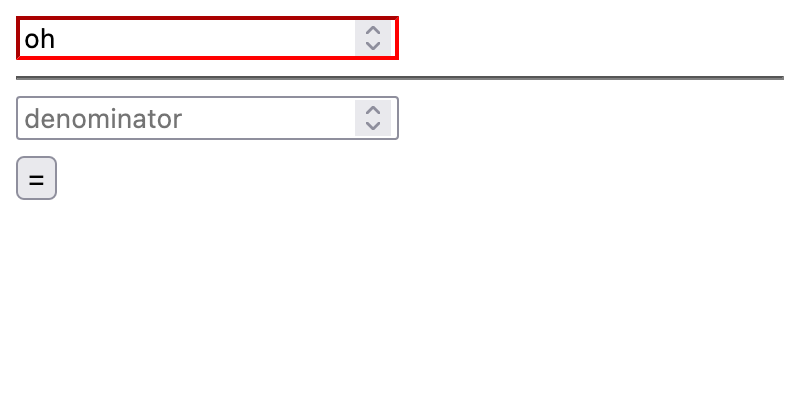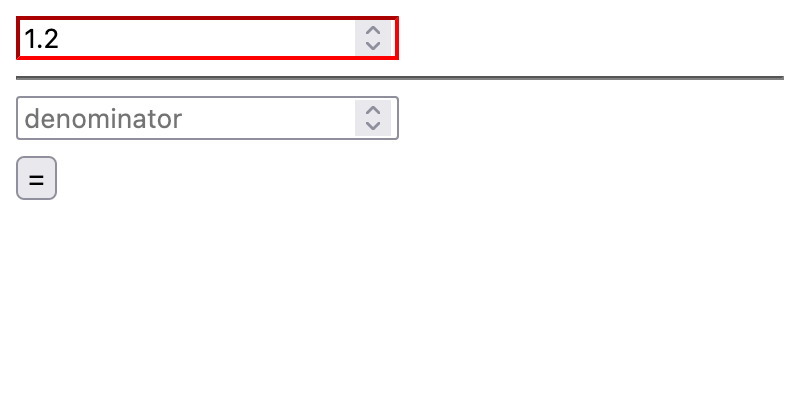## CodeNewbie Community 🌱Marcin Wosinek

Posted on • Originally published at how-to.dev

# Divider App: Improving edge cases

In the previous article, we took a look at all the edge cases one can find in a simple operation: dividing two numbers. Now, let’s improve all those aspects of the application.

Often, the challenge in programming is choosing one of many imperfect solutions. The choice depends on the use cases we want to support in our application. In mathematics, dividing two numbers always gives the same results. In programming, we need to work around the limitations of computers and the data structures. Optimal rounding and the precision we choose depends on the use case. What makes the solution good enough in the context of money would introduce unnecessary errors in scientific calculations. In short: for many questions, there is no perfect solution— just different approaches that fit better or worse into a specific context.

### Rounding

In the previews article, we discussed a number representation issue that causes JS to calculate

``````>> 0.3 / 0.1
2.9999999999999996
``````

This could be solved by rounding to 0.01—which would make perfect sense for currency calculations. In the case of this application, I wanted to maintain support for other use cases as well. For example, consider the division of the numbers orders of magnitudes away from each other, such as `1/1000000`. Overly aggressive rounding would turn those results into 0.

An interesting solution provided by JavaScript is `​​Number.prototype.toPrecision()`. It returns the numbers as a string, limiting the values to provided precision. So

• `0.00012345.toPrecision(4)` returns `"0.0001234"`, and
• `12345.toPrecision(4)` returns `"1.235e+4"`

The first result is perfect; the second is in scientific notation. To turn it back into a standard number, we can use `parseFloat` again—the same function that we use for parsing user input. The final code to run the calculation is thus:

``````const resultValue = numeratorValue / denominatorValue;

result.innerHTML = parseFloat(resultValue.toPrecision(4)).toString();
``````

### Input limitations

Allowing an overly wide range of values is a sure way of generating many edge cases. For example, we have seen in the previous article that numbers above `9007199254740991` start to behave weirdly. Luckily, most of the meaningful uses don’t require such a high number. In our app, we can limit the input values to ± 1 million. This input validation can be implemented with `min` & `max` arguments on the inputs:

``````<input
type="number"
id="denominator"
placeholder="denominator"
min="-1000000"
max="1000000"
/>
``````

## User experience improvements

Once the bugs are resolved, let’s improve the user experience (UX). There are many small details that impact an application’s smoothness and ease of use. Often, the issues are not visible until you have a working interface and you can see how you or the users try to use it. This was the case in our first improvement:

### Support enter

When I was testing the app, intuitively, I tried doing the calculation by pressing enter. Because the calculation was done upon a “click” event, pressing enter had no effect. To add support for the key, I had to change a few things in the application:

• I defined `divide` function, so it can be reused in multiple event callbacks,
• I added an event callback for `keydown` events, and
• in the `keydown` callback, I divide only when the key pressed is `Enter`

Here’s the relevant part of the code after those changes:

``````function divide() {
const numeratorValue = parseFloat(numerator.value),
denominatorValue = parseFloat(denominator.value);

const resultValue = numeratorValue / denominatorValue;

result.innerHTML = resultValue;
}

if (e.key === "Enter") {
divide();
}
});

``````

### Show input validity

Another important part of UX is providing necessary feedback to the user as quickly as possible. We use `<input type=”number” />`, which comes with native input validation: when a user provides a non-number in the input, the field will look empty for us on the JS side. The validation state of the field is available to us as an `:invalid` pseudo-class in CSS. We can use this to style the application:

``````input:invalid {
border-color: red;
}
``````

The results look like:### Allow fraction inputs

Another small issue that appeared in testing is that by default, number validation expects numbers to be integers. For example:To address this hitch, we need to set the `step` attribute on inputs—either to a number to indicate a precision of the input, or to `any` to allow all numbers. Updated code:

``````<input
type="number"
id="denominator"
placeholder="denominator"
min="-1000000"
max="1000000"
step="any"
/>
``````

### Show error messages

As a final improvement of this iteration of the app, let’s show some error messages when inputs cannot be processed. To cover all the possible cases, which are as follows,

• nominator is valid, denominator is corrupt,
• nominator is corrupt, denominator is valid,
• both are corrupt, and
• both are valid,

I needed some slightly complicated code:

``````  const numeratorValue = parseFloat(numerator.value),
denominatorValue = parseFloat(denominator.value);

let errors = [];

if (isNaN(numeratorValue)) {
errors.push("numerator");
}

if (isNaN(denominatorValue)) {
errors.push("denominator");
}

if (errors.length === 0) {
// … calculations
} else {
result.innerHTML = `Cannot parse \${errors.join(" and ")} as number.`;
}
``````

## Complete code

So finally, the `index.html` looks like this:

``````<html>
<meta http-equiv="content-type" content="text/html; charset=utf-8" />
<title>Divider App</title>
<style>
#equals {
margin: 8px 0;
}

input:invalid {
border-color: red;
}
</style>

<body>
<input
type="number"
id="numerator"
placeholder="numerator"
min="-1000000"
max="1000000"
step="any"
/>

<hr />

<input
type="number"
id="denominator"
placeholder="denominator"
min="-1000000"
max="1000000"
step="any"
/>

<br />

<button id="equals">=</button>

<div id="result"></div>

<script src="./main.js"></script>
</body>
</html>
``````

And `main.js`:

``````const body = document.querySelector("body"),
numerator = document.querySelector("#numerator"),
denominator = document.querySelector("#denominator"),
equals = document.querySelector("#equals"),
result = document.querySelector("#result");

function divide() {
const numeratorValue = parseFloat(numerator.value),
denominatorValue = parseFloat(denominator.value);

let errors = [];

if (isNaN(numeratorValue)) {
errors.push("numerator");
}

if (isNaN(denominatorValue)) {
errors.push("denominator");
}

if (errors.length === 0) {
const resultValue = numeratorValue / denominatorValue;

result.innerHTML = parseFloat(resultValue.toPrecision(4)).toString();
} else {
result.innerHTML = `Cannot parse \${errors.join(" and ")} as number.`;
}
}

if (e.key === "Enter") {
divide();
}
});